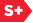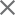### Proceedings Paper

A comparison of localization effects in one-dimensional disordered photonic bandgap structures
Format Member Price Non-Member Price
PDF \$17.00 \$21.00

Paper Abstract

This paper presents a comparison of one-dimensional optical localization effects for both a disordered quarter-wave stack and disordered non-quarter-wave stack. Optical localization in these one-dimensional photonic bandgap structures is studied using the transfer matrix formalism, where each matrix is a function of one or more random variables. As the random matrix product model tends to infinity, Furstenberg's theorem on products of random matrices tells us that the upper (and positive) Lyapunov exponent exists and is deterministic. This Lyapunov exponent is clearly identified as the localization factor (inverse localization length) for the disordered photonic bandgap structure. The Lyapunov exponent can be calculated via the Wolf algorithm which tracks the growth of a vector propagated by the long chain of random matrices. Numerical results of the localization factor are provided using the Wolf algorithm. In the randomized models, layer thicknesses are randomized, being drawn from both a uniform probability density function and a binary probability mass function. Significant notches are noted for a number of the results. The Lyapunov exponent can also be found from Furstenberg's integral formula, which involves integration with respect to the probability distribution of the elements of the random matrices, and the so-called invariant probability measure of the direction of the vector propagated by the long chain of random matrices. This invariant measure can be determined numerically from a bin counting technique similar to the Wolf algorithm. Invariant measure plots based on the bin counting method are shown at selected frequencies.

Paper Details

Date Published: 10 September 2010
PDF: 12 pages
Proc. SPIE 7754, Metamaterials: Fundamentals and Applications III, 775423 (10 September 2010); doi: 10.1117/12.860876
Show Author Affiliations
Glen J. Kissel, Univ. of Southern Indiana (United States)
Cody A. McGill, Univ. of Southern Indiana (United States)

Published in SPIE Proceedings Vol. 7754:
Metamaterials: Fundamentals and Applications III
Allan D. Boardman; Nader Engheta; Mikhail A. Noginov; Nikolay I. Zheludev, Editor(s)